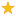quizzes  Forums# Volume of Solid FIgures

created by Simmy8 on 17 Aug 12Medium level (78% of success)    9 questions - 404 players
Volume = lwh1
Find the volume of a rectangular prism. The length is 7 inches, the width is 9 inches, the height is 4 inches.

2
Find the volume of a rectangular prism. The height is 4. 9 cm, the width is 5. 2 cm, the length is 3. 8 cm.

3
Find the length of a rectangular prism. The volume is 252 feet ^3, the height is 7 feet, the width is 6 feet.

4
Find the volume of a cube with length 5 m.

5
FInd the height of a rectangular prism. The volume is 24 cm^3, the width is 2 cm, the length is 4 cm.

6
Find the volume of a cube shaped shipping box with length of 8 inches.

7
Find the volume of a toy box with length 2. 1 feet, width 2. 2 feet, and height 2. 3 feet

8
Find the width of a rectangular prism. The volume is 72 m^3, the height is 2m, the length is 6m.

9
Find the volume of a rectangular prism. The height is 16 cm, the width is 20 cm, the length is 25 cm.

Quiz.biz does not certify the quizzes to be reliable, contact Simmy8 !Comment this Quiz
If you want to rank and comment this quizz, login in or create an account !